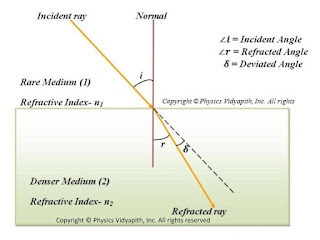### Refraction of light and its Properties

Definition of Refraction of light→ When a light goes from one medium to another medium then light bends from the path. This bending of the light phenomenon is knowns as the refraction of light.

When light goes from a rarer medium to a denser medium, light bends toward the normal as shown in the figure below:Propagation of light from rarer medium to denser medium
When light goes from a denser medium to a rarer medium, the light goes away from normal as shown in the figure below.Propagation of light from denser medium to rarer medium

Properties of refraction of light→

1. The incident ray, normal ray, and refracted ray lie on the same point.
2. According to Snell's law - The ratio of the sine of the incident angle to the sine of the refractive angle is always constant. This constant value is known as the refractive index of the medium.

 $\frac{sin \: i}{sin \: r}=constant(_{1}n_{2})$

$\frac{sin \: i}{sin \: r}= (_{1}n_{2})$

$\frac{sin \: i}{sin \:r}=\frac{n_{2}}{n_{1}}$

Where $_{1}n_{2}$ is the refractive index of medium $(2)$ with respect to medium $(1)$ .

Absolute refractive index→

The ratio of the speed of light in a vacuum to the speed of light in a medium is called the absolute refractive index of the medium.

$refractive\: index(n)=\frac{speed\: of\:light\: in\: vacuum(c)}{speed \:of \:light\: in\: medium(v)}$

 $n=\frac{c}{v}$

Note

According to Snell's law -

$\frac{sin \: i}{sin \: r}=\frac{n_{2}}{n_{1}} \quad\quad (1)$

$\frac{sin \: i}{sin \: r}=\frac{\frac{c}{v_{1}}}{\frac{c}{v_{2}}} \quad\quad \left \{ \because n=\frac{c}{v} \right \}$

$\frac{sin i}{sin r}=\frac{v_{1}}{v_{2}} \qquad (2)$

When the light goes from one medium to another medium frequency of the light does not change but the wavelength of the light changes. So above given equation can be written as

$\frac{sin \: i}{sin \: r}=\frac{\nu \lambda_{1}}{\nu \lambda_{2}} \quad\quad \left \{ \because v=\nu \lambda \right \}$

$\displaystyle \frac{sin \: i}{sin \: r}=\frac{\lambda_{1}}{ \lambda_{2}} \qquad (3)$

From equation $(1)$ ,equation $(2)$, and equation $(3)$ we can write the equation

 $\frac{sin \: i}{sin \: r}=\frac{n_{2}}{n_{1}} =\frac{v_{1}}{v_{2}}= \frac{\lambda_{1}}{ \lambda_{2}}$

Conditions for a light ray to pass undeviated on refraction of light→

A ray of light passes undeviated from medium $1$ to medium $2$ in either of the following two conditions:

1. When the angle of incidence at the boundary of two media is zero $\angle i =0^{\circ} so \ \angle r= 0^{\circ}$.
2. When the refractive index of the medium (2) and medium (1) is the same i.e. $i=r$.

Factors affecting the refractive index of the medium→

The refractive index of a medium depends on basically following three factors:

1. Nature of the medium (on the basis of speed of light)→ Less the speed of light in the medium as compared to that in the air more is the refractive index of the medium. $\left ( n=\frac{c}{v}\right)$

Speed of light in glass- $v_{glass} = 2\times 10^{6} ms^{-1}$

$\mu_{glass} = 1\cdot 5$

Speed of light in water- $v_{water} = 2\cdot25\times10^8ms^{-1}$

$\mu_{water} = 1\cdot33$

2. Physical conditions such as temperature→ With the increase in temperature, the speed of light in the medium, so the refractive index of the medium decreases.

3. The colour or wavelength of light→ The speed of light of all colours is the same in the air ( or vacuum), but in any other transparent medium, the speed of light is different for different colours. In a given medium the speed of red light is maximum and that of the violet light is least, therefore the refractive index of that medium is maximum for violet is and least for red light i.e. $\left ( \ n_{violet}> n_{red}\right )$. The wavelength of visible light increase from violet to red end, so the refractive index of a medium decreases with the increases in wavelength.